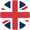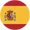# Conjugación del verbo inglés to giveto give ➔dar

## Indicative

I give
you give
he gives
we give
you give
they give

I am giving
you are giving
he is giving
we are giving
you are giving
they are giving

I gave
you gave
he gave
we gave
you gave
they gave

I was giving
you were giving
he was giving
we were giving
you were giving
they were giving

I have given
you have given
he has given
we have given
you have given
they have given

### Present perfect progressive/continuous

I have been giving
you have been giving
he has been giving
we have been giving
you have been giving
they have been giving

### Future

I will/shall give
you will give
he will give
we will/shall give
you will give
they will give

### Future progressive/continuous

I will/shall be giving
you will be giving
he will be giving
we will/shall be giving
you will be giving
they will be giving

### Future perfect

I will/shall have given
you will have given
he will have given
we will/shall have given
you will have given
they will have given

### Future perfect continuous

I will/shall have been giving
you will have been giving
he will have been giving
we will/shall have been giving
you will have been giving
they will have been giving

## Conditional

### Simple

I would/should give
you would give
he would give
we would/should give
you would give
they would give

### Progressive

I would/should be giving
you would be giving
he would be giving
we would/should be giving
you would be giving
they would be giving

### Perfect

I would/should have given
you would have given
he would have given
we would/should have given
you would have given
they would have given

### Perfect progressive

I would/should have been giving
you would have been giving
he would have been giving
we would/should have been giving
you would have been giving
they would have been giving

Otros verbos